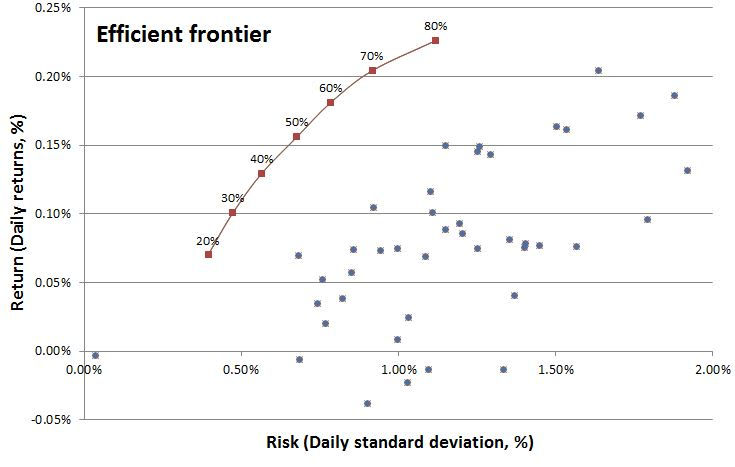top of page
Search
• Henri Caron

# Optimal Portfolio Theory - Applied

With this graph, I want to show the actual benefits of optimising asset allocation, and how it positively affects my portfolio's risk, while maintaining equal returns.

The blue dots represent the individual stocks of my investment universe (Equities in sectors of Technology, Automation, Advanced Materials, see my biography for more information), with their respective risk (average daily volatility) versus return (average daily returns), over the past year.

The red dots represent optimal portfolios according to Markowitz Optimal Portfolio Theory, and as such the optimal combination of the underlying stocks (blue dots), with respectively target annual returns of 20%, 30%, 40%, ... Although the maximum allocation of one individual asset in my actual portfolio is 5%, I applied a maximal allocation in each of the calculated portfolios in this example at 10%, because it better emphasised/visualised the effects I wanted to illustrate. The red line represents the efficient frontier, and is a combination of all optimal portfolios in between the calculated ones.An optimal portfolio is what you want to invest in as a diversified investor, because at that point, you get either the minimal volatility/risk for a target return, either a maximum return for a given volatility/risk level. The volatility of individual assets offset on another (This effect can be calculated through covariance) and as a result, risk is minimised, while equal returns can be achieved. I wanted to visualise that significant reduction in volatility, and hence risk, that can be achieved by properly optimising asset allocation. All of this while maintaining equal returns. I have been applying this theory on my portfolio since its conception, and I am happy about the results.